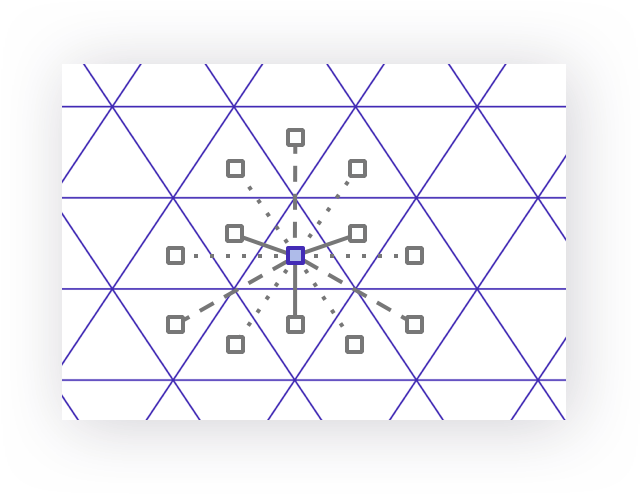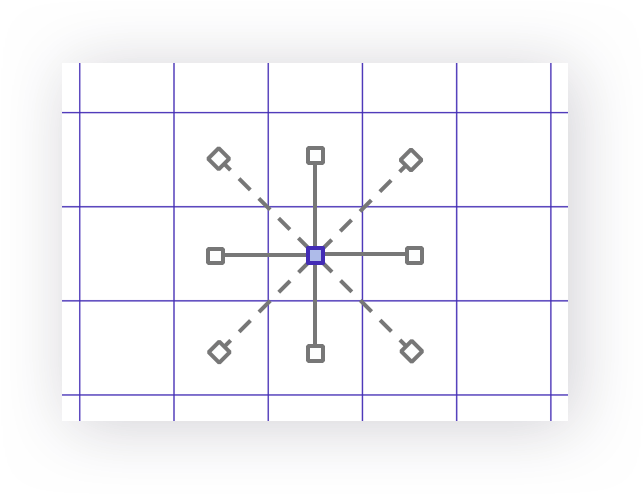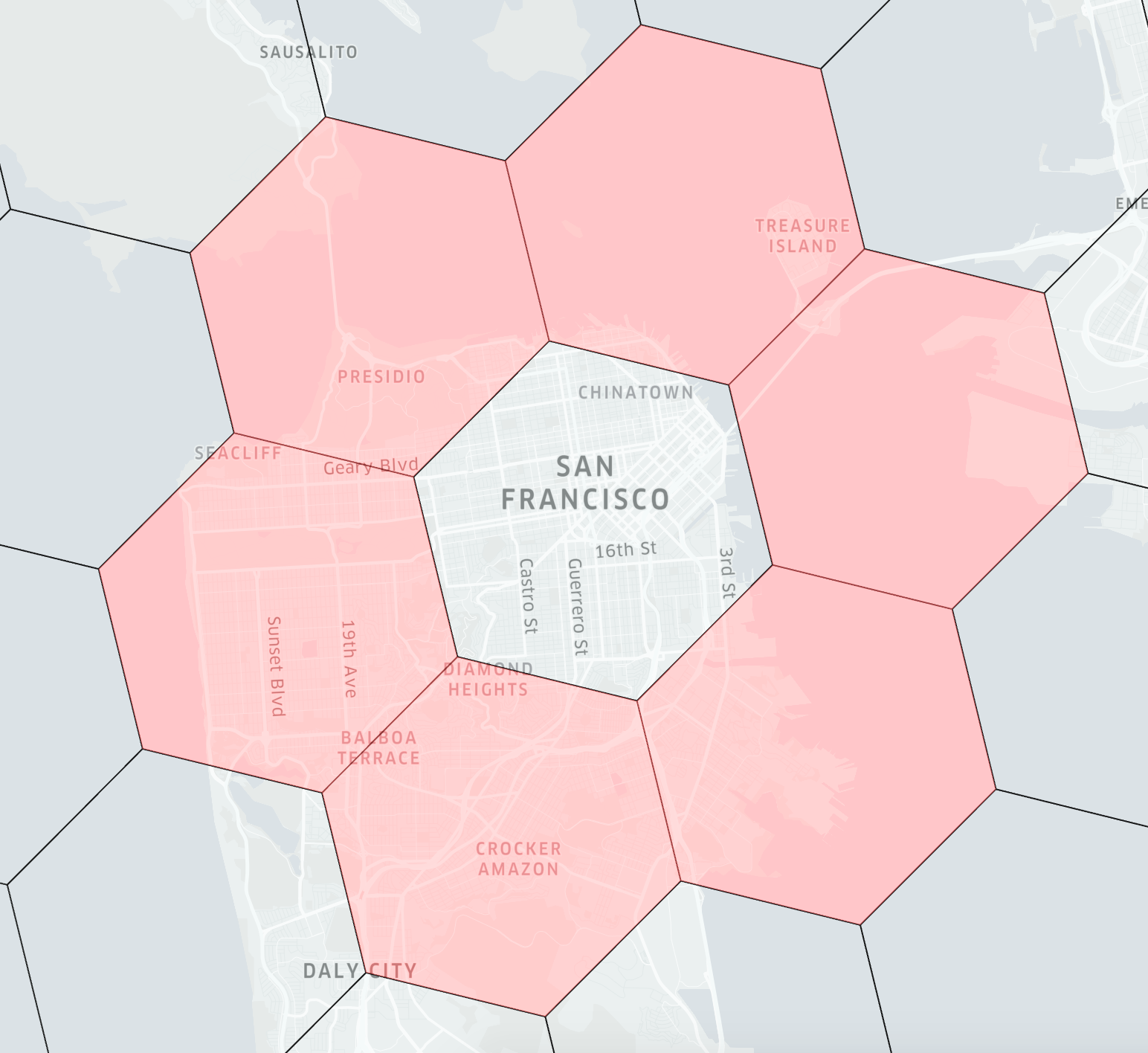Version: 4.x

# Aggregation

Analysis of location data, such as locations of cars in a city, can be done by bucketing locations. (Sahr et al., 2003) Using a regular grid provides smooth gradients and the ability to measure differences between cells.

The cell shape of that grid system is an important consideration. For simplicity, it should be a polygon that tiles regularly: the triangle, the square, or the hexagon. Of these, triangles and squares have neighbors with different distances. Triangles have three different distances, and squares have two different distances. For hexagons, all neighbors are equidistant.

TriangleSquareHexagonTriangles have 12 neighborsSquares have 8 neighborsHexagons have 6 neighbors

This property allows for simpler analysis of movement. Hexagons have the property of expanding rings of neighbors approximating circles:All six neighbors of a hexagon (ring 1)

Hexagons are also optimally space-filling. On average, a polygon may be filled with hexagon tiles with a smaller margin of error than would be present with square tiles.